Reconstruction of the 2019 Pepperbox Hill formation 1.Draw a circle. Draw and extend the horizontal and vertical centerlines. 2.Construct the "two-points" circle (defined by the two end-points of a centerline) between the center of circle 1 and its righthand intersection with the horizontal centerline. 3.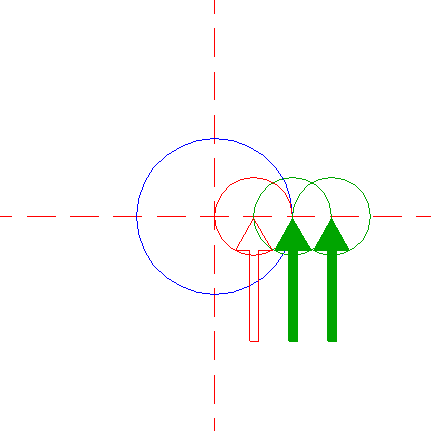Copy circle 2 two times, the first to its righthand intersection with the horizontal centerline, the second to the corresponding intersection of the first. 4.Copy circle 2 to the righthand intersection of the righthand circle 3 and the horizontal centerline. 5.Copy circle 2 two more times, the first to the righthand intersection of circle 4 and the horizontal centerline, the second to the corresponding intersection of the first. 6.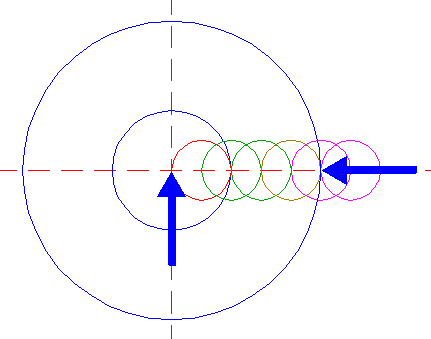Construct a circle concentric to circle 1, tangent to circle 4 at the righthand side. 7.Construct two circles concentric to circle 1, tangent to circles 5 at the righthand sides. 8.Copy circle 6 to the center of circle 4, and move this circle (copy and delete original) to its own righthand intersection with the horizontal centerline. 9.Construct two circles concentric to circle 8, tangent to circles 5 at the righthand sides. 10.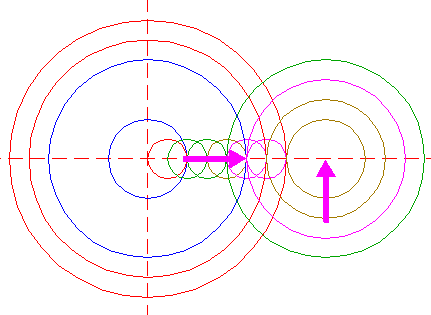Construct a circle concentric to circle 8, tangent to circle 4 at the righthand side. 11.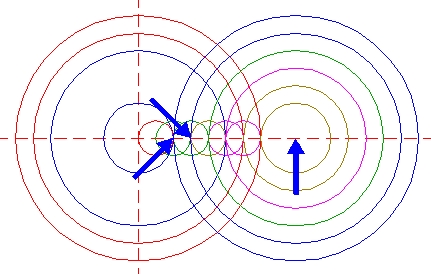Construct two circles concentric to circle 8, passing through the centers of circles 3. 12.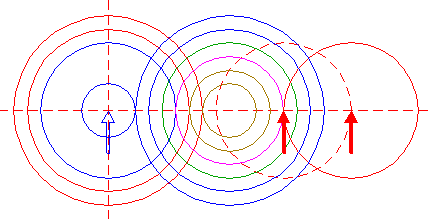Copy circle 6 to the righthand intersection of circle 10 and the horizontal centerline, and move this circle to its own corresponding intersection. 13.Construct two circles concentric to circle 12, tangent to circles 11 at the righthand sides. 14.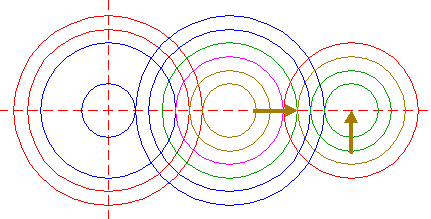Construct a circle concentric to circle 12, tangent to circle 10 at the righthand side. 15.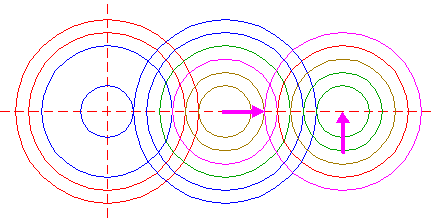Construct a circle concentric to circle 12, tangent to the outer circle 9 at the righthand side. 16.Construct a circle concentric to circle 12, tangent to the inner circle 9 at the righthand side. 17.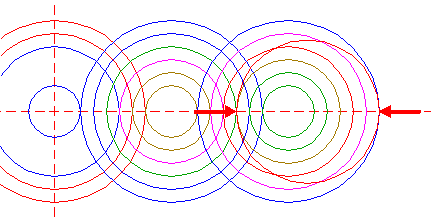Construct a "two-points" circle between the righthand intersections of circles 8 and 16 with the horizontal centerline. 18.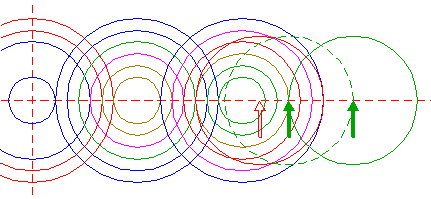Copy circle 17 to the righthand intersection of circle 14 and the horizontal centerline, and move this circle to its own corresponding intersection. 19.Construct a circle concentric to circle 18, tangent to circle 12 at the righthand side. 20.Construct two circles concentric to circle 18, tangent to circles 13 at the righthand sides. 21.Remove all parts from circles 6, 7, 8, 9, 10, 11, 12, 13, 14, 15, 16, 18, 19, and 20, such that... 22.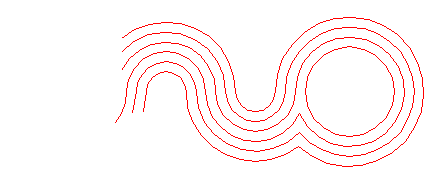...this pattern remains. 23.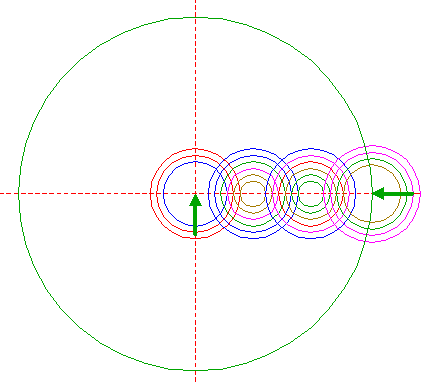Construct a circle concentric to circle 6 (circle 1), passing through the center of circle 18. 24.Construct the inscribed hexagon (regular 6-sided polygon) of circle 23, pointing to the right. 25.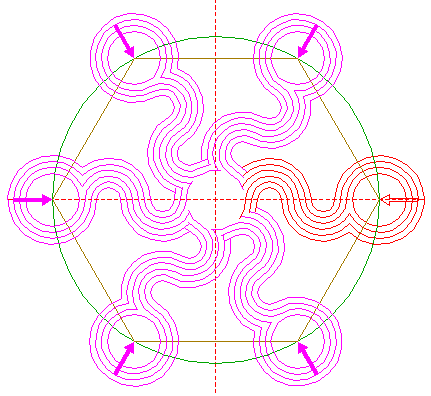Repeat all necessary steps to copy pattern 22 five times, with respect to the other angular points of hexagon 24, while rotating around its center, as shown. 26.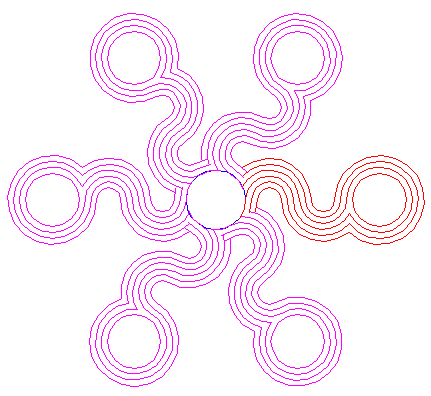Circle 6, and patterns 22, and 25, are used for the final reconstruction. 27.Remove all parts not visible within the formation itself. 28.Colour all areas corresponding to standing... 29.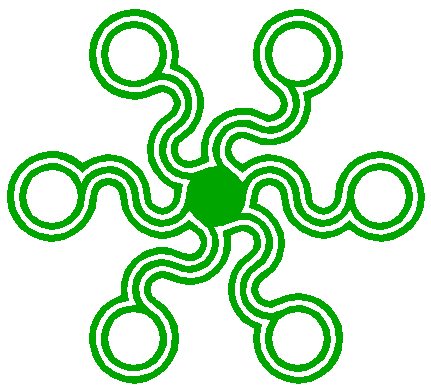...or to flattened crop, and finish the reconstruction of the 2019 Pepperbox Hill formation. 30.The final result, matched with the aerial image.